# Stochastic RSI And ATR Indicator

In the previous blog, we covered the different types of Stochastic Oscillators and went through a few basic strategies based on them. However, we did not cover an indicator which can be called a combination of two indicators, which is the Stochastic RSI indicator. In addition to it, we will also cover another indicator, the ATR indicator and learn how it can be used along with a few other popular indicators.

We will cover the following topics in this article:

## Stochastic RSI

Chande and Kroll, the creators of Stochastic RSI said that on its own, the RSI indicator would usually stay between the ‘overbought’ and ‘oversold’ levels of 80 and 20 and hence, not generate a lot of buy or sell signals.

Thus, they proposed to create an indicator of an indicator by first calculating the RSI values and then applying the stochastic oscillator formula on it.

### How does Stochastic RSI work?

1. Calculate the RSI values with a 14 day period.
2. Then find the Highest RSI value and the Lowest low RSI value
3. Use the modified stochastic oscillator formula ie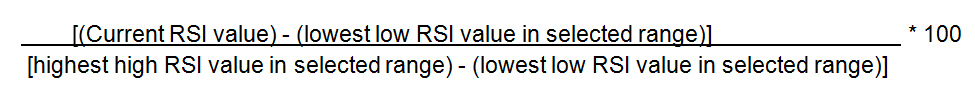To use the same data as we have used for the Stochastic Indicator, the table would be as follows,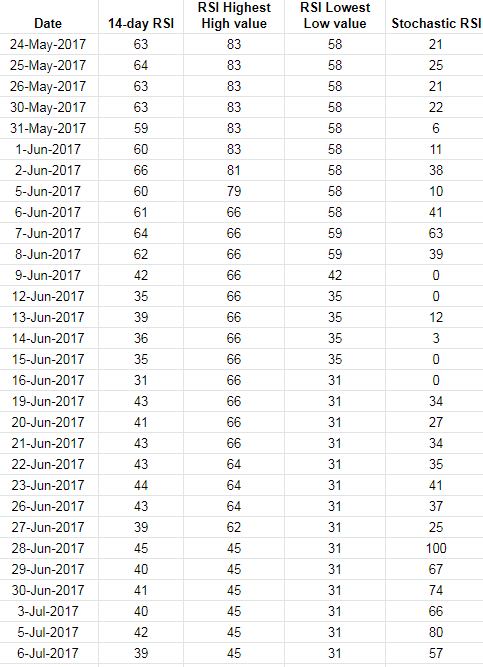Let’s say, for the 14-day period (24 May - 13 June), the Stochastic RSI value would be calculated as follows,= (39 - 35)/(66 -35) * 100 = (4/31)* 100 = 12.

If you plot the RSI vs the Stochastic RSI graph, it would be as follows,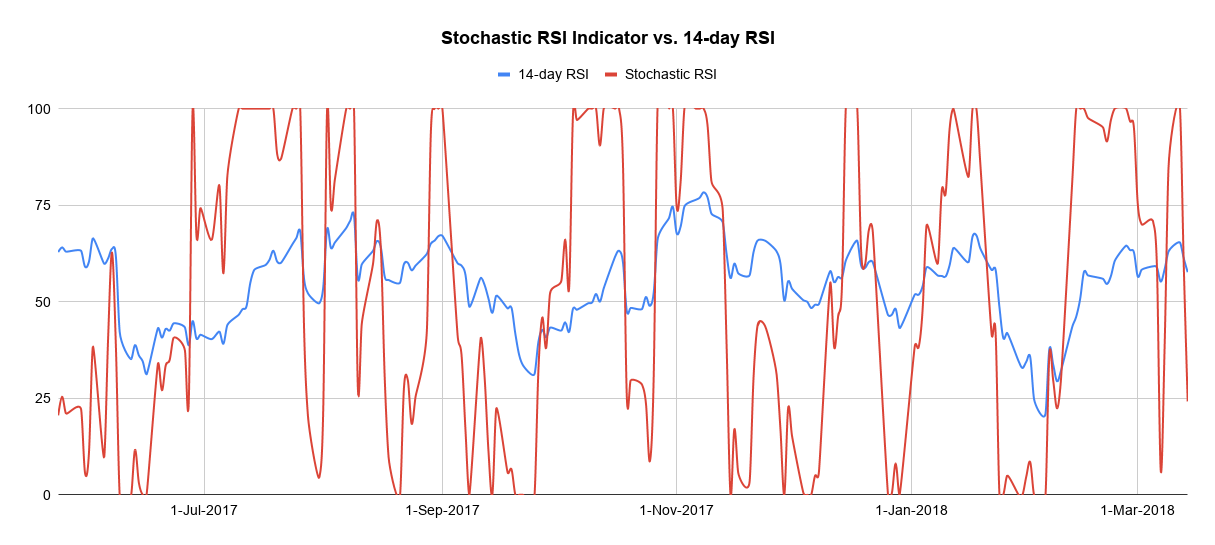As you can see, the Stochastic RSI indicator gives more signals than normal RSI indicator. Due to the sensitivity of the Stochastic RSI, it can be used to identify short-term trends and thus, short term traders could benefit from this indicator.

## Limitations of Stochastic RSI

While the Stochastic RSI was developed to improve the sensitivity of the indicator, it makes the indicator jump from overbought to oversold and vice-versa pretty quickly and thus, generate confusion among traders.

Another downside of the Stochastic RSI is that being an indicator of an indicator, it is two steps away from the price, and hence, it could become out of sync with the current market price.

A plot of the Stochastic RSI with respect to the closing price is shown below for reference.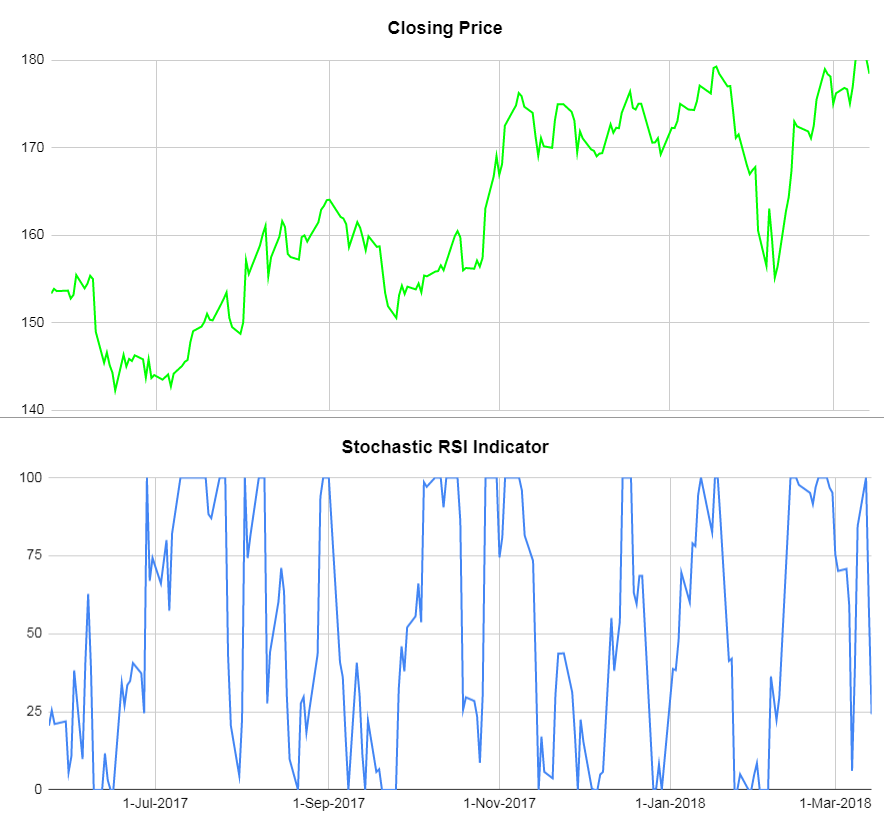Let us know in the comments if you can form an opinion on the direction the price would go based on the graph given above.

We have reiterated at the start of this article that while an indicator is a good way to gauge the market, sometimes using a few indicators together is a good idea. In that respect, we will now cover the Average True Indicator which helps us in making more informed trades and usually ups our confidence level.

## Average True Range (ATR) indicator definition

The reason why ATR is included in this article is two-fold: The ATR is not an indicator which can be used by itself but more as a means of validation for your observations. Thus, it instils confidence in your trading strategy. The second reason is that it works well with other indicators.

One should note that ATR captures only the volatility and not the direction of the market. Let’s see in the next section how we can find the ATR of an asset.

## ATR calculation

Before we calculate the Average true range, we first deduce the true range of an asset.
It is calculated as the maximum value from the following three components:

1. (High - low) of the current day
2. (Today’s high - yesterday’s closing price) and
3. (Yesterday’s closing price - Today’s low)

Do note that for (2) and (3), we use absolute values.

To give you an example: the True range will be calculated in the following manner.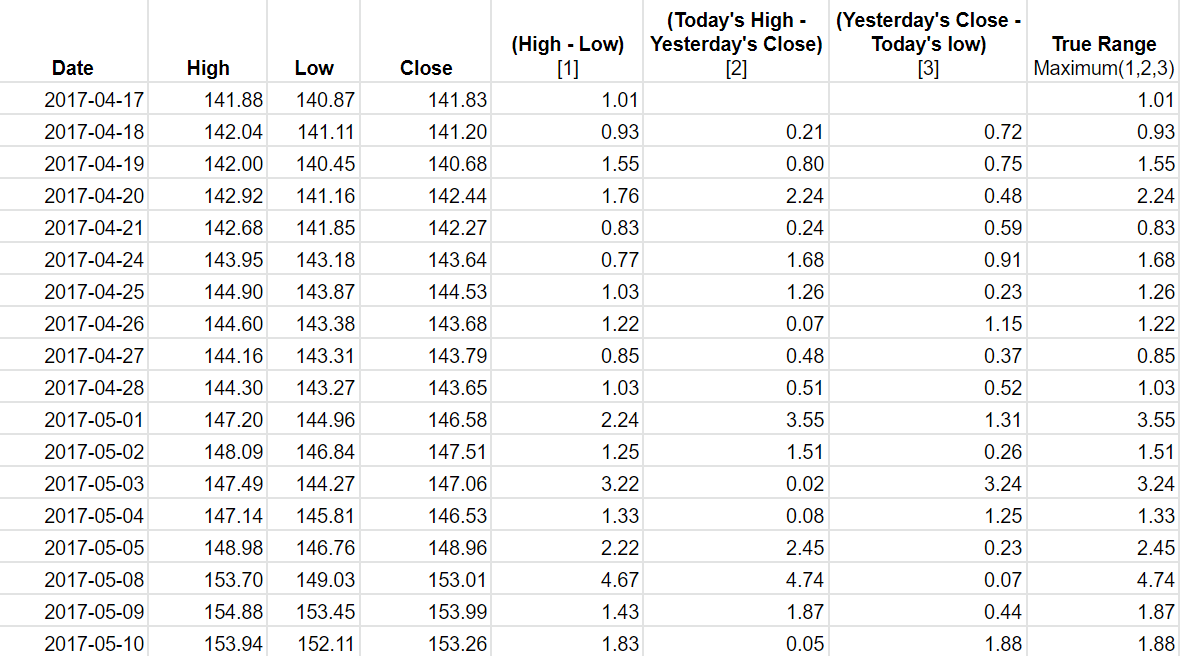The reason we pick the maximum value out of the three values is that it helps us to capture the missing volatility which would have been ignored if we had only taken the high and low values of the present day to calculate the ATR.

Once we have calculated the True range of the Asset, we use a 14 day period to calculate the Average True Range of the asset.

### Formula to calculate ATR

Current value of ATR = [(Previous value of ATR * 13) + Current value of True Range)] / 14.

Thus, the ATR of the asset would be as follows: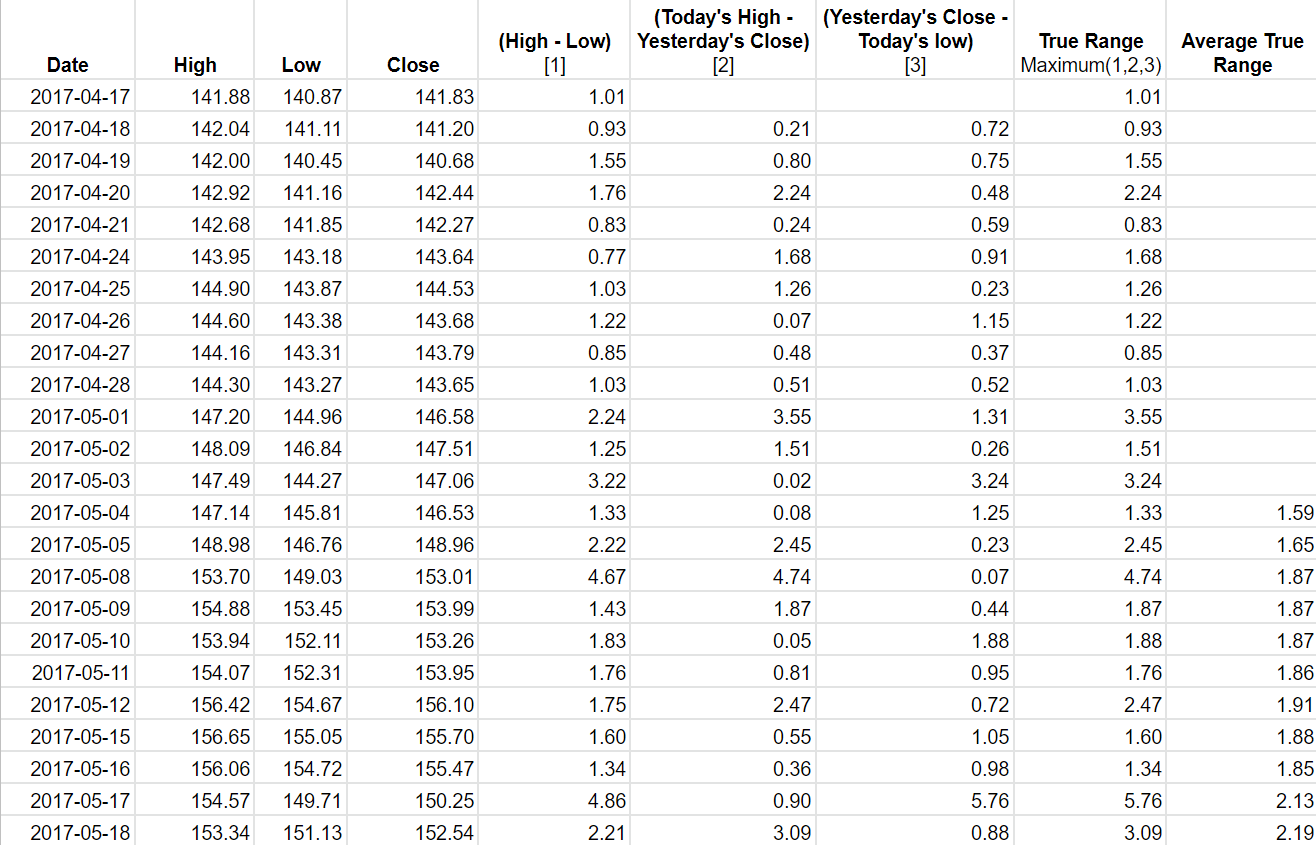The graph for the ATR with respect to the closing price would be in this manner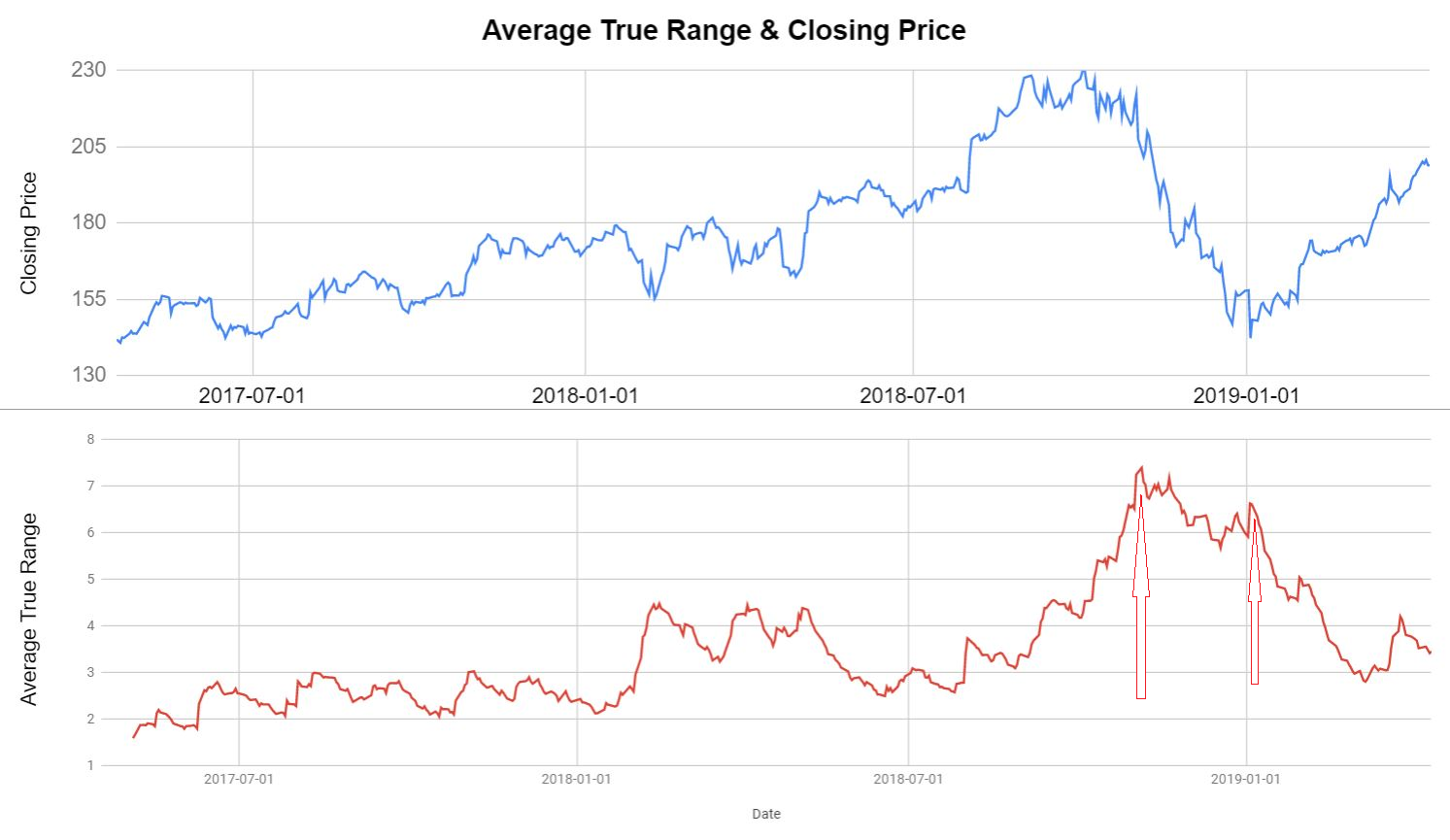You can see that the ATR peaked when:

1. The Closing price started declining sharply
2. The Closing price started increasing.

Thus, the ATR is seen as confirmation that a trend reversal is going to happen.

### ATR and Stochastic Oscillator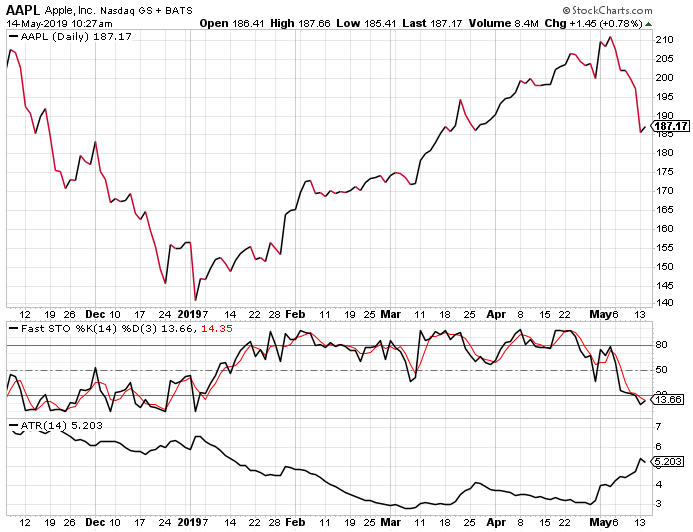You can see that in the graph above, in the first week of January 2019, %K crosses the %D line indicating the reversal of a trend. To support this trend, the ATR is also high during the same time period. Thus, the closing price picks up later on.

## Limitation of ATR

One has to understand that ATR is not used to predict the direction of the price, instead, it shows us the volatility of that particular asset. In that respect, one has to be careful when forming an opinion based on the ATR values alone. A common approach is to compare the ATR with the previous values to get an understanding of the trend of the market and of course, use it in conjunction with the other indicators such as Stochastic oscillators, RSI or MACD.

## Conclusion

In summary, we have gone through the definitions as well as the calculation of the Stochastic indicators as well as the ATR indicator. We also saw a few strategies which can be used with the help of these indicators. Technical indicators do help us in understanding the market better and make informed trades. You can learn more about indicators as well as their applications in the following learning track: Algorithmic Trading for Everyone

Disclaimer: All investments and trading in the stock market involve risk. Any decisions to place trades in the financial markets, including trading in stock or options or other financial instruments is a personal decision that should only be made after thorough research, including a personal risk and financial assessment and the engagement of professional assistance to the extent you believe necessary. The trading strategies or related information mentioned in this article is for informational purposes only.